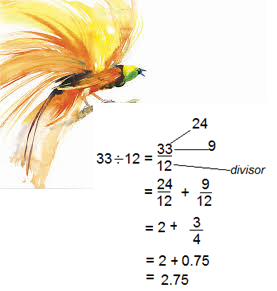# Do Mental Maths Fast with Four Tips

Students and maths enthusiasts can do mental maths fast with these tips. The strategies for addition and subtraction, and multiplication and division are useful when dealing with large numbers.

It may need MORE practice to perfect the skills you may need to do mathematics in classroom, examination questions and use generally in life.

### Recommended: PNG Mathematics Past Papers Download

The four mathematics skills can help you to do maths accurately when

• subtracting,
• multiplying and
• dividing.

## 1. Easy Adding: Mental Maths Fast

Tip: Separating numbers into parts, then add

Here is the first example | how to do mental addition by partitioning

Evaluate: 52 + 27 + 23 + 18How to do mental addition by partitioning | PNG MATHS

If you can see parts like 20, 20 and 10 (add to 50) and the other 50 make an easy 100.

Also, 7 and 3 (=10) and 8 and 2 (=10) gives 20.

By doing this you can get 120 (100 and 20) without having to use a calculator.

Practice

1. 37 + 42 + 18 + 14

2. 47 + 53 + 73

## 2. Easy subtracting: Mental Maths Fast

Tip: Make the last digits the same (353 and 33 see e.g. below), then subtract in parts.

Here is an example to put clarity to this abstract idea | Reducing to zero by parts and simplify.

Evaluate: 353 – 35Reducing big numbers to zero and simplify mentally | PNG MATHS

Practice

1. 133 – 35

2. 257 – 99

## 3. Easy Multiplying: Mental Maths Fast

Tip: Separate one number (usually the smaller number) into parts, multiply each out, then add.

This method is helpful when multiplying bigger numbers such as by 10s, 100s and 1000s.

A good skill to have early in Grades 8 and 10 where the examinations require the students to use mental calculation.

Here is an illustrated example | Multiplying by place value reduction and addition.

Evaluate: 27 × 13

Practice

1. 57 × 13

2. 43 × 17

## 4. Easy dividing: Mental Maths Fast

Tips: Identify a multiple of the divisor (e.g. 24 is a multiple of 12 closest to 33, see e.g. below), and simplify

This is a good technique as it relates to the addition of fraction. Though a tricky concept (addition of fractions), if you know it well, you could do the division of large numbers well too.

Here is the example | Dividing by identifying common fractions, add them and simplify.

Evaluate: 33 ÷ 12Dividing by identifying common fractions, add them and simplify

Practice

1. 37 ÷ 12

2. 75 ÷ 14

*You can leave your answers in mixed fraction instead of decimal.

## Mental arithmetic and challenges

There are several ways of adding, subtracting, multiplying and dividing large numbers when dealing with classroom questions by doing Mental Maths Fast.

Some methods are more traditional approaches, whilst others are different ways of doing the same thing.

Perhaps, what is important is that some methods may not be readily employed in certain practical situations when a need arises. Therefore, many modern teachers tend to use mastery approaches to problem-solving in classrooms. Moreso, the teachers in advanced educational settings prefer using mental arithmetic methods that their students can use in real life.

The four methods above, are tips for mental manipulation of numbers both in classrooms and in real life.

## PNG Classroom mathematics approaches

Many changes are happening in PNG’s education system. Change in structure (in 2016) and change in the curriculum (2015, OBE to SBE) are two significant educational changes. The changes must be equally complimented by good learning content (syllabus).

Methods above are examples of creating effective learning contents, especially when introducing mathematics in Grades 7 – 8 and Grades 9 – 10.

Traditional mathematics and practical mathematics are still grey areas in PNG mathematics syllabus. The examples above are illustrations of mathematics students can do when introduced well at early Grade Levels mentioned above.

## Global best practices and mathematics in PNG classrooms

PNG mathematics teaching has to be more problem-solving related. If we are to make a nation of quick thinkers, we’ve got to

• identify classroom mathematics that is often use in real life;
• develop practical mathematics skills;
• introduce the approaches uniformly and systematically;
• instruct mathematics teachers to include the practical mathematics approaches in their termly plans; and
• relate the lessons for everyday use – not just to help students in tests and exams.

## Application of mathematics ideas and problem-solving approaches

The above methods are simply examples of how mathematics in the classroom can be streamlined to prepare students for life. The current PNG lower (and upper) secondary school mathematics syllabi and teachers’ guides have the right intention (intended/conceptualised) ideas.

But, what’s lacking in areas of problem-solving and mental arithmetic is a clear and precise activity and teaching strategy that is uniform. By this I mean, well-developed and implemented for students across the country.

Importantly, the development of mathematics learning and approaches to teaching mathematics should adopt global best practices. This will give students the best opportunity to master numbers early in life.

### Comments and share. You can also check out the work on algebra.

This work was first published on PNG INSIGHT BLOG